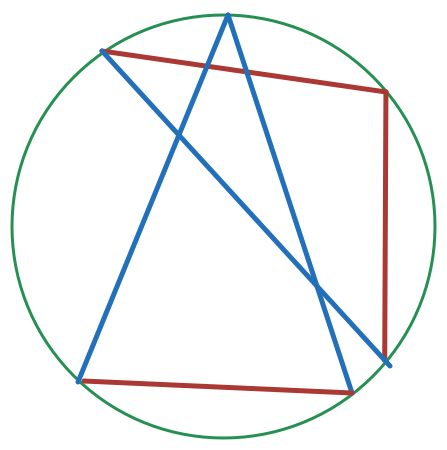# Isosceles Triangles in a Circle

Geometry Level 5An isosceles triangle with base $a$ and the lateral side $b$, and an isosceles triangle with base $b$ and the lateral side a are inscribed in a circle of radius $R$, given that $a \neq b$, then evaluate $\frac{ab}{R^{2}}$ .

###### You can try more of my Questions here .
×

Problem Loading...

Note Loading...

Set Loading...